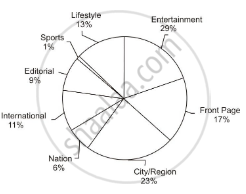# What Percentage (Approximately) More than the Print Space Allocation for 'Editorial' is the Print Space Allocation for 'Front Page'? - Mathematics

MCQ

Direction:  Answer the question on the basis of the information given below.

The pie chart given below shows the percentage of print space allocation of articles printed on different sections of a newspaper.What percentage (approximately) more than the print space allocation for 'Editorial' in the print space allocation for 'Front Page'?

• 150%

• 50%

• 100%

• 90%

#### Solution

90%

Explanation:

The correct answer is 90%, because the percentage of print space allocation for 'Editorial' is 9% and that for Front Page is 17%. So the space allocation for Front Page is more than that for Editorial by :

(17 - 9)/9 xx 100 = 88.88 % which is closest to 90%.

Concept: Graph and Charts (Entrance Exam)
Is there an error in this question or solution?
Share